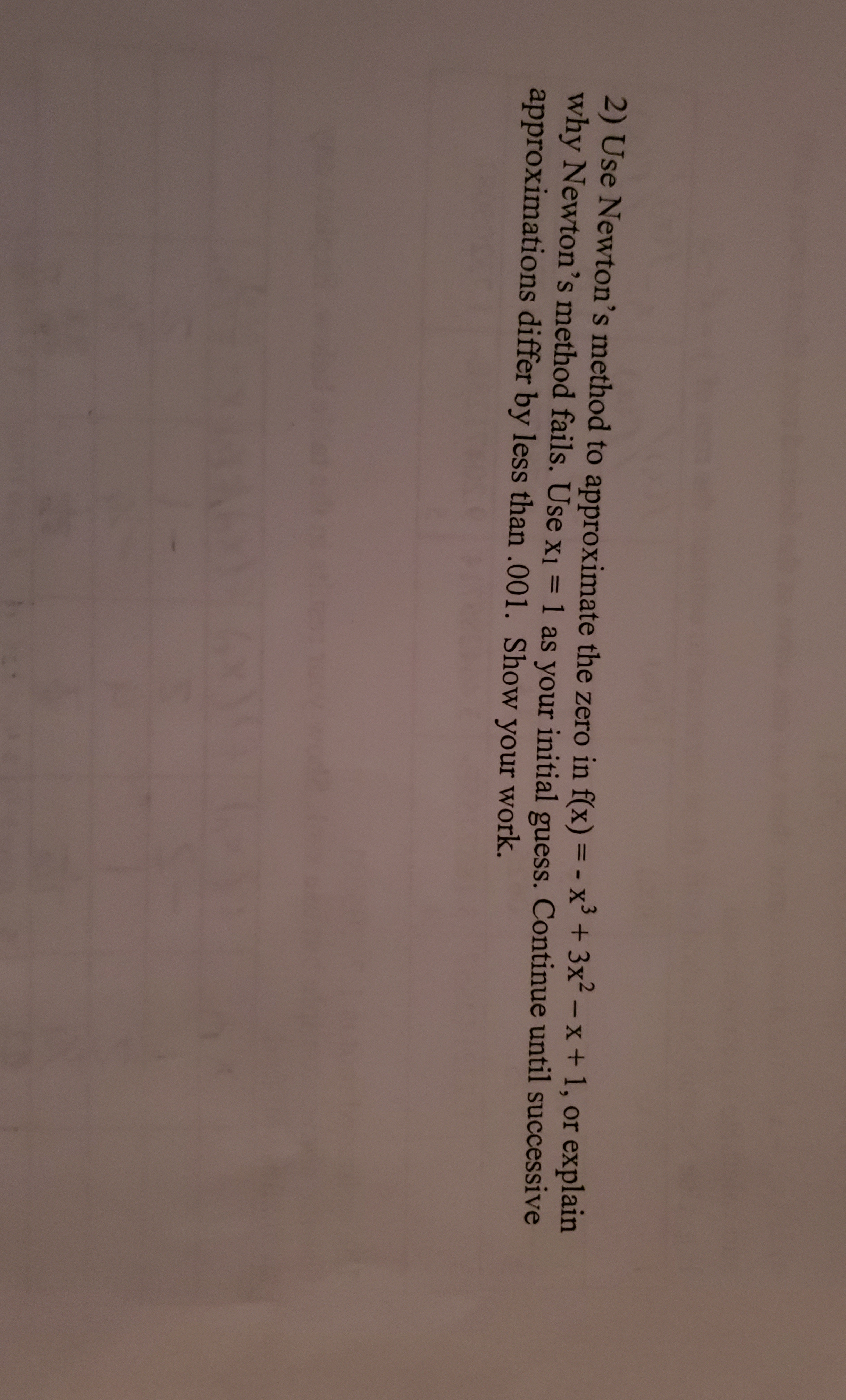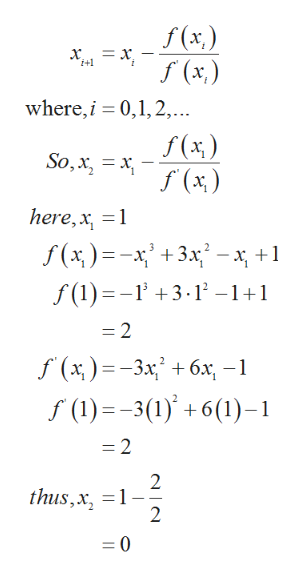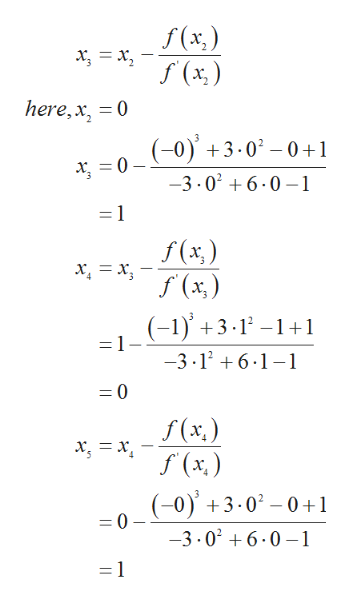# 2) Use Newton's method to approximate the zero in f(x) = - x3 + 3x-x + 1, or explainwhy Newton's method fails. Use x1 = 1 as your initial guess. Continue until successiveapproximations differ by less than .001. Show your work

Question
39 viewshelp_outlineImage Transcriptionclose2) Use Newton's method to approximate the zero in f(x) = - x3 + 3x-x + 1, or explain why Newton's method fails. Use x1 = 1 as your initial guess. Continue until successive approximations differ by less than .001. Show your work fullscreen
check_circle

Step 1

Consider the given function,

Step 2

According to the Newton’s method,help_outlineImage Transcriptionclosef (x) = x, 1+1 where,i 0,1, 2,... f (x) f (x) So,x x here,x 1 f (1) 3.12 -1+1 =2 f (x)3x 6x f (1)=-3(1)+6(1)-1 =2 2 =1 2 thus,x =0 fullscreen
Step 3

The next iteration val...help_outlineImage Transcriptionclosef(x) f (x) here,x 0 (-0) 3.02-0+1 0 -3.026.0-1 -1 f(x) f (x) (-1)3.12-1+1 =1- -3.126.1-1 0 f(x.) (x.) (-0)3.02-0 +1 = 0 -3.026.0-1 - 1 fullscreen

### Want to see the full answer?

See Solution

#### Want to see this answer and more?

Solutions are written by subject experts who are available 24/7. Questions are typically answered within 1 hour.*

See Solution
*Response times may vary by subject and question.
Tagged in

### Calculus